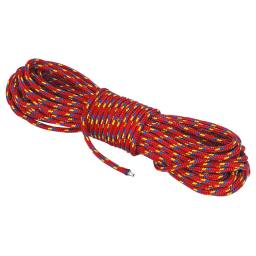# Rope

One half of rope use Mam for packaging, half of the rest got son, half of the rest he took father and two fifths of what remained, got daughter. Remained 143 cm of rope.

How long was rope at the beginning?

Correct result:

x =  1907

#### Solution:We would be pleased if you find an error in the word problem, spelling mistakes, or inaccuracies and send it to us. Thank you!Tips to related online calculators
Do you have a linear equation or system of equations and looking for its solution? Or do you have quadratic equation?
Do you want to convert length units?

## Next similar math problems:

• Simple equation 8Solve the following equation: 36=-(1+7x)-6(-7-x)
• Unknown numberIdentify unknown number which 1/5 is 40 greater than one tenth of that number.
• Simple equationSolve the following simple equation: 2. (4x + 3) = 2-5. (1-x)
• Dropped sheetsThree consecutive sheets dropped from the book. The sum of the numbers on the pages of the dropped sheets is 273. What number has the last page of the dropped sheets?
• Simple equationSolve for x: 3(x + 2) = x - 18
• Simple equation 1035= 7*3*x what is x?
• Equation 29Solve next equation: 2 ( 2x + 3 ) = 8 ( 1 - x) -5 ( x -2 )
• Simple equation 9Solve the following equation: -8y+5=-9y+9
• Equation?
• EquationSolve the equation: 1/2-2/8 = 1/10; Write the result as a decimal number.
• Equation 11Solve equation: ?
• Find xSolve: if 2(x-1)=14, then x= (solve an equation with one unknown)
• Negative in equation2x + 3 + 7x = – 24, what is the value of x?
• CakesGrandmother baked cakes. Half of its was poppy, quarter with plum jam and 16 cheesecakes. How many cakes she baked in total?
• FactoryIn the factory workers work in three shifts. In the first inning operates half of all employees in the second inning and a third in the third inning 200 employees. How many employees work at the factory?
• The math testThe math test contains 20 problems. For each correctly solved problem, the solver gets 3 points, for each incorrectly solved or unsolved problem, 2 points are deducted. Ondrej got 25 points. How many problems did he solve correctly?
• InfirmaryTwo thirds of children from the infirmary went on a trip seventh went to bathe and 40 children remained in the gym. How many children were treated in the infirmary?